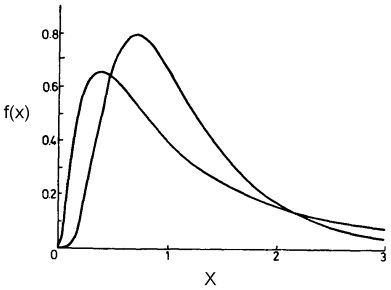The Rocscience International Conference 2021 Proceedings are now available. Read Now

# Lognormal Distribution

If a random variable has a Lognormal distribution, then its natural logarithm has a Normal distribution. This is the meaning of the term Lognormal. Like the Exponential distribution, the Lognormal distribution can only be used for variables which are always positive.

Lognormal probability density functions